Courses

# NCERT Solutions(Part - 3) - Decimals Class 6 Notes | EduRev

## Class 6 : NCERT Solutions(Part - 3) - Decimals Class 6 Notes | EduRev

The document NCERT Solutions(Part - 3) - Decimals Class 6 Notes | EduRev is a part of the Class 6 Course Mathematics (Maths) Class 6.
All you need of Class 6 at this link: Class 6

Exercise 8.5

Question 1: Find the sum in each of the following:

(a) 0.007 + 8.5 + 30.08

(b) 15 + 0.632 + 13.8

(c) 27.076 + 0.55 + 0.004

(d) 25.65 + 9.005 + 3.7

(e) 0.75 + 10.425 + 2

(f) 280.69 + 25.2 + 38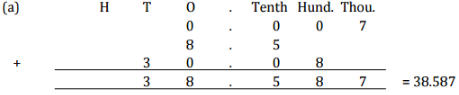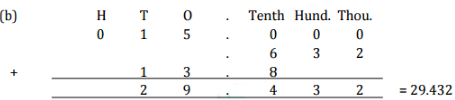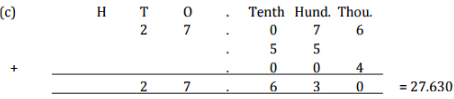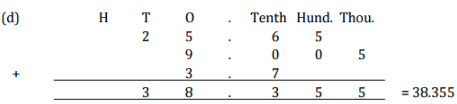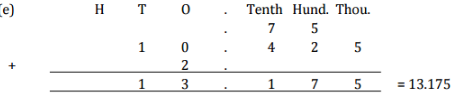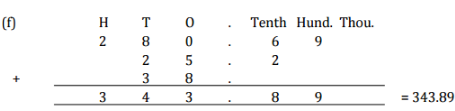Question 2: Rashid spent Rs 35.75 for Maths book and Rs32.60 for Science book. Find the total amount spent by Rashid.

Answer 2: Money spent for Maths book = Rs 35.75

Money spent for Science book = Rs 32.60

Total money spent = Rs 35.75 + Rs 32.60 = Rs 68.35

Therefore, total money spent by Rashid is Rs 68.35.

Question 3: Radhika’s mother have her Rs10.50 and her father gave her Rs15.80. Find the total amount given to Radhika by the parents.

Answer 3: Money given by mother = Rs 10.50

Money given by father = Rs 15.80

Total money received by Radha = Rs 10.50 + Rs 15.80 = Rs 26.30

Question 4: Nasreen bought 3 m 20 cm cloth for her shirt and 2 m 5 cm cloth for her trouser. Find the total length of cloth bought by her.

Answer 4: Cloth bought for shirt = 3 m 20 cm = 3.20 m

Cloth bought for trouser = 2 m 5 cm = 2.05 m

Total length of cloth bought by Nasreen = 3.20 + 2.05 = 5.25 m

Therefore, the total length of cloth bought by Nasreen is 5.25 m

Question 5: Naresh walked 2 km 35 m in the morning and 1 km 7 m in the evening. How much distance did he walk in all?

Answer 5: Distance travelled in morning = 2 km 35 m = 2.035 km

Distance travelled in evening = 1 km 7 m = 1.007 km

Total distance travelled = 2.035 + 1.007 = 3.042 km

Therefore, the total distance travelled by Naresh is 3.042 km.

Question 6: Sunita travelled 15 km 268 m by bus, 7 km 7 m by car and 500 m by foot in order to reach her school. How far is her school from her residence?

Answer 6: Distance travelled by bus = 15 km 268 m = 15.268 km

Distance travelled by car = 7 km 7 m = 7.007 km

Distance travelled on foot = 500 m = 0.500 km

Total distance travelled = 15.268 + 7.007 + 0.500 = 22.775 km

Therefore, total distance travelled by Sunita is 22.775 km.

Question 7: Ravi purchases 5 kg 400 g rice, 2 kg 20 g sugar and 10 kg 850 g flour. Find the total weight of his purchases.

Answer 7: Weight of Rice = 5 kg 400 g = 5.400 kg

Weight of Sugar = 2 kg 20 g = 2.020 kg

Weight of Flour = 10 kg 850 g = 10.850 kg

Total weight = 5.400 + 2.020 + 10.850 = 18.270 kg

Therefore, the total weight of Ravi’s purchase = 18.270 kg.

Exercise 8.6

Question 1: Subtract:

(a) Rs. 18.25 from Rs. 20.75

(b) 202.54 m from 250

(c) Rs. 5.36 from Rs. 8.40

(d) 2.051 km from 5.206 km

(e) 0.314 kg from 2.107 kg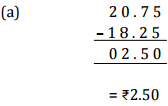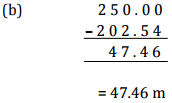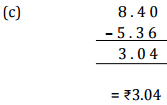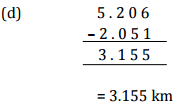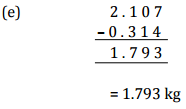Question 2:

Find the value of:

(a) 9.756 – 6.28

(b) 21.05 – 15.27

(c) 18.5 – 6.79

(d) 11.6 – 9.847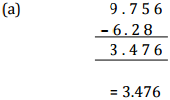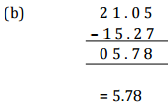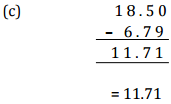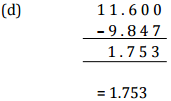Question 3: Raju bought a book of Rs35.65. He gave Rs50 to the shopkeeper. How much money did he get back from the shopkeeper?

Answer 3: Total amount given to shopkeeper = Rs50

Cost of book = Rs35.65

Amount left = Rs50.00 – Rs35.65

= Rs14.35

Therefore, Raju got back Rs14.35 from the shopkeeper.

Question 4: Rani had Rs18.50. She bought one ice-cream for Rs11.75. How much money does she have now?

Answer 4: Total money = Rs18.50

Cost of Ice-cream = Rs11.75

Amount left = Rs18.50 – Rs11.75

= Rs6.75

Therefore, Rani has Rs6.75 now.

Question 5: Tina had 20 m 5 cm long cloth. She cuts 4 m 50 cm length of cloth from this for making a curtain. How much cloth is left with her?

Answer 5: Total length of cloth = 20 m 5 cm = 20.05 m

Length of cloth used = 4 m 50 cm = 4.50 m

Remaining cloth = 20.05 m – 4.50 m = 15.55 m

Therefore, 15.55 m of cloth is left with Tina.

Question 6: Namita travels 20 km 50 m every day. Out of this she travels 10 km 200 m by bus and the rest by auto. How much distance does she travel by auto?

Answer 6: Total distance travel = 20 km 50 m = 20.050 km

Distance travelled by bus = 10 km 200 m = 10.200 km

Distance travelled by auto = 20.050 – 10.200 = 9.850 km

Therefore, 9.850 km distance travels by auto.

Question 7: Aakash bought vegetables weighing 10 kg. Out of this 3 kg 500 g in onions, 2 kg 75 g is tomatoes and the rest is potatoes. What is the weight of the potatoes?

Answer 7: Weight of onions = 3 kg 500 g = 3.500 kg

Weight of tomatoes = 2 kg 75 g = 2.075 kg

Total weight of onions and tomatoes = 3.500 + 2.075 = 5.575 kg

Therefore, weight of potatoes = 10.000 – 5.575 = 4.425 kg

Thus, the weight of potatoes is 4.425 kg

Offer running on EduRev: Apply code STAYHOME200 to get INR 200 off on our premium plan EduRev Infinity!

## Mathematics (Maths) Class 6

185 videos|229 docs|43 tests

,

,

,

,

,

,

,

,

,

,

,

,

,

,

,

,

,

,

,

,

,

;